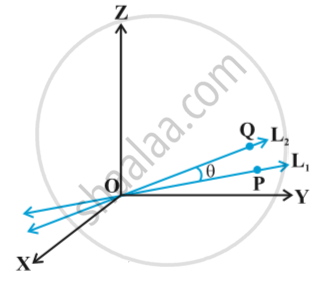# Angle Between Two Lines

#### notes

Let L_1 and L_2 be two lines passing through the origin and with direction ratios a_1, b_1, c_1 and a_2, b_2, c_2, respectively. Let P be a point on L_1 and Q be a point on L_2. Consider the directed lines OP and OQ as given in following fig.Let θ be the acute angle between OP and OQ. Now recall that the directed line segments OP and OQ are vectors with components a_1, b_1, c_1 and a_2, b_2, c_2, respectively. Therefore, the angle θ between them is given by
 cos θ = |(a_1a_2 + b_1b_2 + c_1c_2)/(sqrt(a_1^2 + b_1^2 + c_1^2) sqrt (a_2^2 + b_2^2 + c_2^2))|     ...(1)
The angle between the lines in terms of sin θ is given by
sin θ = sqrt (1- cos^2 θ)

= sqrt (1- (a_1a_2 + b_1b_2 + c_1c_2)^2 / ((a_1^2 + b_1^2 + c_1^2) (a_2^2 + b_2^2 + c_2^2)))

= sqrt ((a_1^2 + b_1^2 + c_1^2) (a_2^2 + b_2^2 + c_2^2) - (a_1a_2 + b_1b_2 + c_1c_2)^2 ) / (sqrt(a_1^2 + b_1^2 + c_1^2 )  sqrt(a_2^2 + b_2^2 +c_2^2))

= sqrt ((a_1 b_2 - a_2 b_1) ^2 + (b_1 c_2 - b_2 c_1)^ 2 + (c_1 a_2 - c_2 a_1) ^2 )/ (sqrt (a_1^2 + b_1^2 + c_1^2) sqrt (a_2^2 + b_2^2 + c_2^2))  ...(2)

If instead of direction ratios for the lines L_1 and L_2, direction cosines, namely, l_1, m_1, n_1 for L_1 and l_2, m_2, n_2 for L_2 are given, then (1) and (2) takes the following form:
cos θ = |l_1 l_2 + m_1m_2 + n_1n_2|  ("as"  l_1^2 + m_1^2 + n_1^2 = 1 = l_2^2 + m_2^2 + n_2^2)  ...(3)
and sin θ = sqrt( (l_1m_2 - l_2m_1)^2 - (m_1n_2 - m_2n_1)^2 + (n_1l_2 - n_2 l_1)^2)   ..(4)

Two lines with direction ratios a_1, b_1, c_1 and a_2, b_2, c_2 are
(i) perpendicular i.e. if θ = 90° by (1)
a_1a_2 + b_1b_2 + c_1c_2 = 0
(ii) parallel i.e. if θ = 0 by (2)
a_1/a_2 = b_1/b_2 = c_1/c_2
Now, we find the angle between two lines when their equations are given. If θ is acute the angle between the lines
vec r = vec a_1 + lambda vec b_1 and vec r = vec a_2 + mu vec b _2
then cos theta = |(vec b _1 . vec b_2)/(|vec b _1|| vec b _2|)|`

If you would like to contribute notes or other learning material, please submit them using the button below.

### Shaalaa.com

Three Dimensional Geometry Part 3 - Straight line [00:35:32]
S
0%